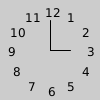# Py5Vector.rotate()#

Rotate vector by a specified angle.

## Examples#def setup():
py5.translate(py5.width / 2, py5.height / 2)
py5.text_align(py5.CENTER, py5.CENTER)
v = py5.Py5Vector(0, 40)
py5.fill(0)
for i in range(1, 13):
v.rotate(-py5.TWO_PI / 12)
py5.text(f'{i}', v.x, -v.y)
py5.line(0, 0, 0, -30)
py5.line(0, 0, 20, 0)def setup():
py5.size(100, 100, py5.P3D)
py5.translate(10, 20, -20)
# rotate so the viewer can better see the shape
py5.rotate_z(0.5)
py5.rotate_y(-0.5)
v2 = py5.Py5Vector(60, 0, -30)
with py5.begin_shape(py5.TRIANGLE_FAN):
py5.vertex(0, 0, 0)
for i in range(16):
v2.rotate(-py5.TWO_PI / 15, dim='x')
py5.vertex(v2.x, v2.y, v2.z)


## Description#

Rotate vector by a specified angle. This method is only applicable to 2D and 3D vectors. Use the angle parameter to specify the rotation angle. To rotate 3D vectors, you must use the dim parameter to specify which dimension to rotate around. The dimension can be specified with the values 1, 2, or 3, or by using the strings 'x', 'y', or 'z'.

A 2D vector will be rotated in the counter-clockwise direction for positive angle values and in the clockwise direction for negative angle values.

A 3D vector’s rotation will follow the right-hand rule. Using your right hand, point your thumb in the direction of the axis to rotate around. Your fingers will curl in the direction of rotation when the angle parameter is positive.

## Signatures#

rotate(
angle: float,  # angle of rotation, measured in radians
) -> Py5Vector2D

rotate(
angle: float,  # angle of rotation, measured in radians
dim: Union[int, str],  # dimension to rotate around
) -> Py5Vector3D


Updated on March 06, 2023 02:49:26am UTC